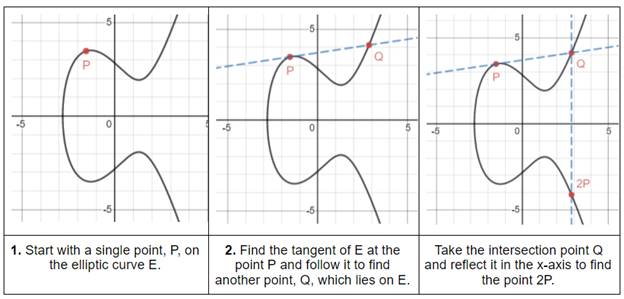top of pageSearch

# Elliptic Curve Cryptography

By Gemma Penson - Computer Science Student @ Trinity Hall, Cambridge

Cryptography is the action of keeping data secure when it’s being transmitted over a network. This is achieved by ensuring that only you and your intended recipient can read the messages that you send. If you are unfamiliar with cryptography, please take a look at the article “Cryptography: Keeping Information Secret” (linked at the end of the article) for a brief introduction on the fundamentals of this mathematical practice.

The RSA cryptosystem is a security method for sending messages over a network. It uses the principle that, whilst multiplying prime numbers together is relatively straightforward, factorising a number into its prime components is mathematically tricky. When it was introduced in 1977 it was considered to be a highly secure method of communication, but over time algorithms such as Quadratic Sieve were created which can efficiently factorise primes. These new algorithms mean that a user’s private key can be calculated from their freely available public key and the maximum key value used in the communication which has led to a decline in RSA’s security and thus its use today.

The creation of RSA prompted researchers to explore other mathematical-based functions that could be used to create a computationally secure cryptosystem. In 1985, one such system was proposed based on an obscure branch of mathematics known as elliptic curves.

An elliptic curve is a finite set of points satisfying an equation in two variables, one with degree two and the other with degree three, where a variable’s degree is the highest exponent (power) it occurs with. One such equation that meets this requirement is in the form:All elliptic curves are naturally a mathematical group: a set of elements together with an operation (called the group law) that combines any two elements to form a third. An example of a group is the set of integers together with the addition operator. For elliptic curves, this group law is constructed using an elliptic curve property which states that any non-vertical straight line will intersect the curve in at most three places. You can therefore take two points on the curve and the group law known as the direct sum in order to find another point on the curve. Finding direct sums is the underlying operation in elliptic curve cryptography and can be performed as follows:This final, reflected point is known as the direct sum of P and Q on the curve E. It’s often written as P ⊕ Q, where ⊕ is the mathematical symbol for a direct sum. In the example above, two different points, P and Q, were summed but in elliptic curve cryptography single points are summed with themselves. In 2D the line which is used in step two is the only line that goes through both P and Q but multiple lines will go through a single point - which line do you use?When calculating the direct sum on an elliptic curve you should be aware of a special case which occurs when you take the direct sum of two points that are equal in magnitude but opposite in sign:Similarly to RSA, in elliptic curve cryptography the range of an elliptic curve must be restricted where any point whose coordinate components (x and y) do not lie in this range must be “wrapped around” until they do. This range is imposed by a maximum number which must be prime in order to ensure that the graph has good cryptographic properties. In addition, only points with integer coordinates are used in the cryptosystem as it would be incredibly tricky to work with every possible point on a curve.

Below is an example of a curve whose range has been restricted to integer coordinate components below a prime maximum value. If you look closely you can still see the horizontal symmetry in the modified graph - it still carries the same geometric properties that it had before being processed.The direct sum of points on the modified graph can then be efficiently computed by a machine in order to create the cryptosystem. If the lines used to calculate the direct sum hit the borders of the graph without hitting a point then they will be wrapped to the opposite side of the graph:We’ve now covered all of the required pieces to form the elliptic curve cryptosystem! An elliptic curve cryptosystem (EEC) is defined by picking an elliptic curve equation, a public point on the curve and a maximum value that must be prime. The public key can be found by finding the sum of the public point and itself (P⊕ P) k times, where a is then the private key.

Computing the private key from the public key uses a function known as the elliptic curve discrete logarithm function, which is an incredibly effective cryptography function as it makes it extremely difficult to work out the private key. Despite almost three decades of research, mathematicians still haven't found an algorithm to solve the elliptic curve discrete logarithm that improves upon a naive brute-force approach.

Algorithms harnessing elliptic curves are rapidly gaining momentum as using ECC over RSA saves time, power and computational resources. Today EEC is used for a variety of purposes, from protecting internal connections in the U.S. government to assuring online anonymity with the Tor project.